Test: KCL & KVL in AC Circuits - 1

# Test: KCL & KVL in AC Circuits - 1 - Electrical Engineering (EE)

Test Description

## 10 Questions MCQ Test GATE Electrical Engineering (EE) 2024 Mock Test Series - Test: KCL & KVL in AC Circuits - 1

Test: KCL & KVL in AC Circuits - 1 for Electrical Engineering (EE) 2023 is part of GATE Electrical Engineering (EE) 2024 Mock Test Series preparation. The Test: KCL & KVL in AC Circuits - 1 questions and answers have been prepared according to the Electrical Engineering (EE) exam syllabus.The Test: KCL & KVL in AC Circuits - 1 MCQs are made for Electrical Engineering (EE) 2023 Exam. Find important definitions, questions, notes, meanings, examples, exercises, MCQs and online tests for Test: KCL & KVL in AC Circuits - 1 below.
Solutions of Test: KCL & KVL in AC Circuits - 1 questions in English are available as part of our GATE Electrical Engineering (EE) 2024 Mock Test Series for Electrical Engineering (EE) & Test: KCL & KVL in AC Circuits - 1 solutions in Hindi for GATE Electrical Engineering (EE) 2024 Mock Test Series course. Download more important topics, notes, lectures and mock test series for Electrical Engineering (EE) Exam by signing up for free. Attempt Test: KCL & KVL in AC Circuits - 1 | 10 questions in 30 minutes | Mock test for Electrical Engineering (EE) preparation | Free important questions MCQ to study GATE Electrical Engineering (EE) 2024 Mock Test Series for Electrical Engineering (EE) Exam | Download free PDF with solutions
 1 Crore+ students have signed up on EduRev. Have you?
Test: KCL & KVL in AC Circuits - 1 - Question 1

### An AC voltage is given by v(t) = 5 + sin(2000πt), what is the time period and the dc component in v(t)?

Detailed Solution for Test: KCL & KVL in AC Circuits - 1 - Question 1

Concept

For a DC signal

• The average value over one time period is non zero
• The non-zero value is known as the DC value
•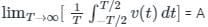• 'A 'is the DC component of the signal

Calculation

Given the signal v(t) = 5 + sin(2000πt)

Now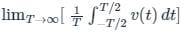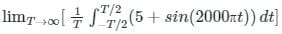we have,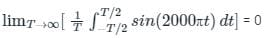The average value of sinusoidal signal over one time period is always 0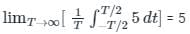Hence the DC component of the signal v(t) is 5

Now observe the AC component in signal v(t)

sin(2000πt) is the AC component

The angular frequency ω = 2000π

Also ω = 2π / T ; here T is the Time period of the signal

2π / T= 2000π

T = 10-3 s

T =1 ms

The Time period of the signal is 1 ms

Therefore the correct answer is option 4

Test: KCL & KVL in AC Circuits - 1 - Question 2

### If the voltage and current in an A.C. circuit is 90° out of phase, then the power in the circuit will be -

Detailed Solution for Test: KCL & KVL in AC Circuits - 1 - Question 2

The correct answer is 'option C'

Concept:

The power in an AC circuit is given by the expression

ϕP=VIcosϕ........(1)

here, ϕ is the angle between voltage and current.

Solution:

It is given to us that ϕ = 900

From eqn(1)

P = 0 Watts

Test: KCL & KVL in AC Circuits - 1 - Question 3

### In the case of a sinusoidal current, the unit of the amplitude is:

Detailed Solution for Test: KCL & KVL in AC Circuits - 1 - Question 3

Waveform: The curve obtains by plotting the instantaneous value of any electrical quantity such as voltage, current, or power.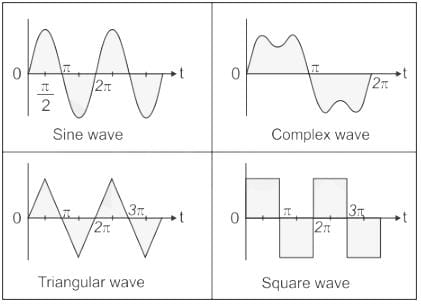Cycle: One complete set of positive and negative values or maximum or minimum value of the alternating quantity is called a cycle.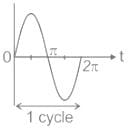Note: One-half cycle of the wave is called Alternation.

Amplitude: the maximum value of the positive or negative alternative quantity is called Amplitude.

• For sinusoidal current, the unit of the amplitude is amperes.
• For sinusoidal voltage, the unit of the amplitude is volts.
• It is also known as peak value or crest value.
• Peak-Peak Value = 2 × Amplitude
• In the given figure amplitude of the waveform is Vm.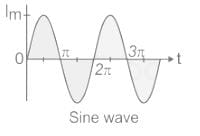Time period (T): It is the time required to complete one cycle. It measured in seconds.

Frequency: The number of cycles completed per second is called frequency.

f = 1 / T

It measured in Hz or radian/sec.

Test: KCL & KVL in AC Circuits - 1 - Question 4

A sinusoid wave is expressed as 5 sin(4πt – 60°). Find the frequency.

Detailed Solution for Test: KCL & KVL in AC Circuits - 1 - Question 4

Concept:

Considered a sinusoidal Alternating wave of voltage and current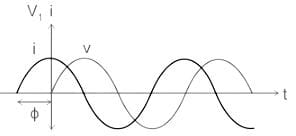From the waveform:

v = Vmsin⁡(ωt)

And,

i = Imsin⁡(ωt + ϕ)

Where Vm and Iare the maximum value of instantaneous voltage and current respectively.

v, i is the instantaneous value of voltage and current at any instant t.

ω is the angular frequency in radian/second.

And, ω = 2πf

f is the frequency in Hz

From the above three equations instantaneous value of voltage and current can be written as:

v = Vmsin⁡(2πft)

And,

i = Imsin⁡(2πft + ϕ)

Calculation:

Sinusoidal expression of the wave is given as 5 sin (4πt – 60°)

Comparing the above expression with the given equation:

as:

v = Vmsin⁡(2πft)

Or,

i = Imsin⁡(2πft + ϕ)

Hence, ω = 4π

We know that,

ω = 2πf

∴ 4π = 2πf

∴ f = 2 Hz

Test: KCL & KVL in AC Circuits - 1 - Question 5

In the circuit shown in the figure, the value of node voltage ��2 is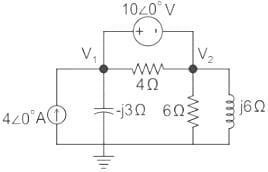Detailed Solution for Test: KCL & KVL in AC Circuits - 1 - Question 5Since there is a voltage source present between the two nodes, the concept of supernode will be applied.

By KCL, using supernode at Node (1) and (2), we can write: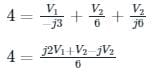24 = j2 V1 + (1 - j) V2      ---(1)

By KVL, V1 – V2 = 10 ∠0°

V1 = V2 + 10       ---(2)

Substituting (2) in (1), we get:

24 = j2 (V2 + 10) + (1 - j) V2

24 = j2 V2 + j20 + (1 - j) V2

24 = j20 + (1 + j) V2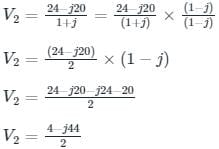V2 = 2 – j22 V

*Answer can only contain numeric values
Test: KCL & KVL in AC Circuits - 1 - Question 6

Three currents i1, i2 and i3 meet at a node as shown in the figure. If i1 = 3 cos (ωt) ampere, i2 = 4 sin (ωt) ampere and i3 = I3 cos (ωt + θ) ampere, the value of I3 in ampere is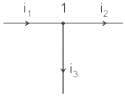Detailed Solution for Test: KCL & KVL in AC Circuits - 1 - Question 6

By applying KCL at node 1,

i1 = i2 + i3

i3 = i1 – i2

= 3 cos ωt – 4 sin ωt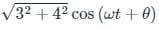= 5 cos (ωt + θ)

Given that, i3 = I3 cos (ωt + θ)

⇒ I3 = 5A.

Test: KCL & KVL in AC Circuits - 1 - Question 7

What will be the period of the sinusoid, v(t) = 12 cos (50t + 30°)?

Detailed Solution for Test: KCL & KVL in AC Circuits - 1 - Question 7

Concept:

Considered a sinusoidal Alternating wave of voltage and current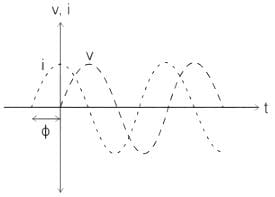From the waveform:

v = Vmsin⁡(ωt)

And,

i = Imsin⁡(ωt + ϕ)

Where Vm and Im are the maximum value of instantaneous voltage and current respectively.

v, i is the instantaneous value of voltage and current at any instant t.

ω is the angular frequency in radian/second.

And, ω = 2πf

f is the frequency in Hz

From the above three equations instantaneous value of voltage and current can be written as:

v = Vmsin⁡(2πft)

And,

i = Imsin⁡(2πft + ϕ)

Calculation:

Sinusoidal wave is given as v = 12 cos (50t + 30°)

Comparing to the given equation

v = Vmsin(ωt ± θ)

ωt = 2πft = 50t

f = 2π50

We know that,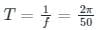=0.1257sec

*Answer can only contain numeric values
Test: KCL & KVL in AC Circuits - 1 - Question 8

In the circuit shown, the current I flowing through the 50 Ω resistor will be zero if the value of capacitor C (in μF) is ______.Detailed Solution for Test: KCL & KVL in AC Circuits - 1 - Question 8

Concept:

Current through an open circuit or through a circuit with impedance tending to infinity is zero.

Calculation:

We are given a circuit shown below: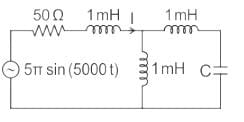The impedance seen from the voltage source is Zeq (say) which can be calculated as: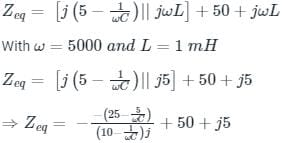For I = 0, Zeq → ∞ --- (1)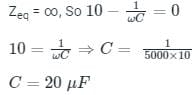Test: KCL & KVL in AC Circuits - 1 - Question 9

Consider a current source i(t) connected across a 0.5 mH inductor, where i(t) = 0 A for t < 0 and i(t) = (8e-250t - 4e-1000t) A for t ≥ 0. The voltage across the inductor at t = 0 s is

Detailed Solution for Test: KCL & KVL in AC Circuits - 1 - Question 9

Concept:

When current source i(t) connected across an inductor(L), then the voltage across the inductor is given as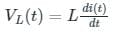When voltage source v(t) connected across a capacitor(C), then the current across the capacitor is given as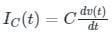Calculation:

Given i(t) = (8e-250t - 4e-1000t) A for t ≥ 0, L = 0.5 mH

Then the voltage across the inductor is given as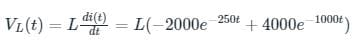At t = 0 its value is given as

VL = 0.5 × 10-3 × (- 2000 + 4000)

VL = 1 V

*Answer can only contain numeric values
Test: KCL & KVL in AC Circuits - 1 - Question 10

Three 400 Ω resistors are connected in delta and powered by a 400 V (rms), 50 Hz balanced, symmetrical R - Y - B sequence, three-phase three-wire mains. The rms value of the line current (in amperes, rounded off to one decimal place) is

Detailed Solution for Test: KCL & KVL in AC Circuits - 1 - Question 10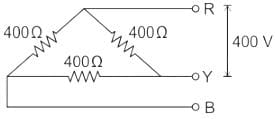In delta connection, VL = Vph = 400 Ω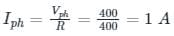ILine = √3 Iphase = √3 × 1 = 1.732 A

## GATE Electrical Engineering (EE) 2024 Mock Test Series

23 docs|285 tests
Information about Test: KCL & KVL in AC Circuits - 1 Page
In this test you can find the Exam questions for Test: KCL & KVL in AC Circuits - 1 solved & explained in the simplest way possible. Besides giving Questions and answers for Test: KCL & KVL in AC Circuits - 1, EduRev gives you an ample number of Online tests for practice

## GATE Electrical Engineering (EE) 2024 Mock Test Series

23 docs|285 tests